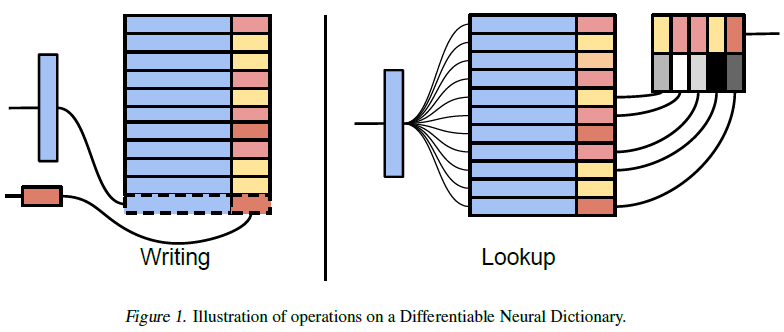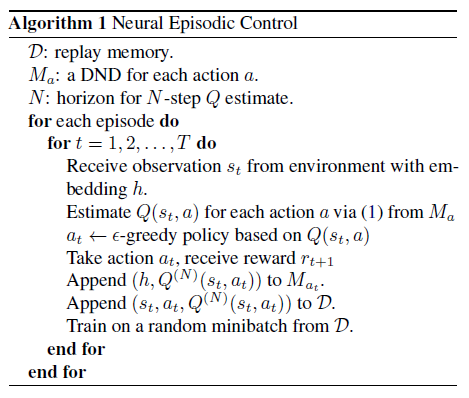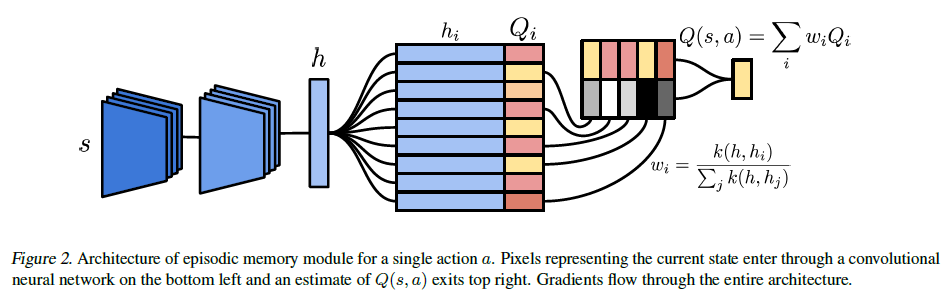# Nerual Episodic Control - Deepmind

NEC 是一种基于能够利用过去经历的值函数半表格表示(semi-representation): 包含有缓慢改变的状态表示(slowly changing state representations)和快速更新的对于这个值函数的更新, 以此更快的适应新的环境.

## 背景介绍

1. SGD 的较小/较大的学习率使得圣经网络训练变得很慢/灾难性的后果
2. 环境中有很少的 instances 时非零的(也就是很稀疏), 因此, 这种情况导致了低回报的样本远远超过了高回报的样本, 使得 agent 很难采取有回报的动作
3. 通过 value-bootstrapping 技术，反馈信号传播，如 Q-learning，导致经过先前与环境交互的历史，反馈信息一次只传播一步。如果变化出现时更新以相反的方向发生，那么这可能非常有效。然而为了训练不相关的小批量 DQN-style，算法在随机选择的状态转移（transition）上训练，并且为了进一步稳定训练，需要使用缓慢更新的目标网络进一步减缓reward 传播。类似于 DQN 的算法都是在经验池中随机(randomly)选择状态转移(minibatch)来使得训练变得稳定, 但也使得 target 网络更新变慢以至于降低了 reward 的传播.

• 解决 DRL 以上的三个问题同时加快在各种环境(指的是 environment, 不是探讨泛化性)的训练速度
• agent 可以立即锁定(latch)那些经历过的非常成功的策略, 而不需要像 A3C 和 DQN 使用 SGD 那样需要通过多次优化.

### DNC

agent 通过在环境中习得的经验的 semi-tabular representation 来拥有一些类似于 episodic memory 的特性: 长时间记忆和 context-based lookup. 而 semi-tabular representation 是一种仅能够附加的将缓慢变化的键和快速更新的值进行绑定的记忆. 并且使用 context-based lookup 在 agent 选择(应该是行为)的时候通过给定的键查找(retrieve)相应的值. 有很多的独特的记忆系统可以引入, 论文中使用了"简化"的 DNC, 论文的结构没有学习如何在 DNC 中写记忆, 因为这会导致学习变慢以及带来的巨大的时间消耗. 相反, 论文中选择把所有的经验写入到记忆中, 而非 DNC 的在每次 episode 结束之后就清空内存.

## NEC 组成

agent 由三部分组成:

• 处理输入状态的像素图像的卷积网络$s$
• 针对每一个行为的记忆系统集合
• 从网络中读取记下来的$Q(s,a)$值

## Differentiable Neural Dictionary• lookup 的操作(键$h$映射到数值值$o$):

$$\begin{equation} o=\sum_iw_iv_i \label{dnd_lookup} \end{equation}$$

DND 的输出是一个所有在记忆中的值函数的加权和, 加权使用的是 normalised kernels between the lookup key and the corresponding key in memory. 为了能够在大规模的记忆中进行查询, 需要限制$\eqref{dnd_lookup}$在p-邻近的范围内(一般p=50);另外我们可以基于kd树的an approximate

nearest neighbours algorithm 来进行查找操作. 在一个 DND 查找结束后, 一个新的键值对被写入记忆中: 其中key是查询的key, key对应的值是需要应用层面来指定(在论文中,使用NEC更新来明确?). 写入操作是仅仅添加到尾部同时重复的键会被更新.

## NEC agent 的结构

agent 的算法结构## 添加 (s,a) 到记忆系统

$$Q^{(N)}(s_t,a)=\sum_{j=0}^{N-1}\gamma^jr_{t+j}+\gamma^N\max_{a'}Q(s_{t+N},a')$$

$$\begin{equation}Q_i\gets{Q_i+\alpha(Q^{(N)}(s,a)-Q_i)}\label{dnd_inner_update}\end{equation}$$

• $\alpha$能够是high value, 允许重复访问过的有一个稳定的表示的状态来更新他们的值估计.(We found that could take on a high value, allowing repeatedly visited states with a stable representation to rapidly update their value function estimate.)
• 在类似于 episode 结束的时候批量更新记忆能够有助于计算的性能(可能是每若干个batch就更新)
• 当DND容量不足的时候,覆盖那些很少出现的邻居.

## 学习

agent 的参数的更新通过L2损失: 预测的对于给定的动作的Q和在回放缓存(只包含$(s_t,a_t,R_t)$)进行随机mini-batch采样的估计. 回放缓存是一个缩小版的DQN的经验池, 所有的元素$(s_t,a_t,R_t)$都是均匀采样生成mini-batch. 所以学习的过程就是如图2的是完全可以微分的以至于可以用对损失函数用梯度下降, BP使用loss的梯度来 更新嵌入的网络(应该就是CNN)权重和bias以及每个action的DND记忆的keys和values;使用比更新查询(queries,$\eqref{dnd_inner_update}$ 其学习率记为$\alpha$)更小的学习率.

# 实验